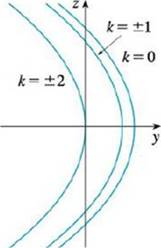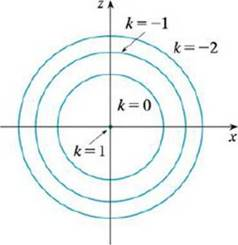Chapter 12.6, Problem 29E

Chapter
Section
Textbook Problem

# Sketch and identify a quadric surface that could have the traces shown.Traces in x = kTraces in y = kTo determine

To sketch and identify: The given quadratic surface.

Explanation

Traces in y=k :

The traces for y=k are the circular traces, which is parallel to the xz plane. These circles are centered at origin point of the xz plane and radii of these circles are increases by decreasing the value of y.

For y=1 , the circular traces seems like a single point, which is occurs on the origin point of the xz plane. This indicates that there is no further traces in y=k for k>1 and the vertex of the curve is (x,y,z)=(0,1,0)

### Still sussing out bartleby?

Check out a sample textbook solution.

See a sample solution

#### The Solution to Your Study Problems

Bartleby provides explanations to thousands of textbook problems written by our experts, many with advanced degrees!

Get Started

#### Find more solutions based on key concepts